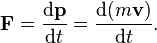# What do the d and p symbols mean in physics

• B
• Quantum Velocity
Quantum Velocity
Hey guy! I've just started learn physics and i ran into a problem.
Can you guy let me know what is d and p mean in thisMentor
d represents the derivative (see Leibniz's notation) while p is momentum (with bold indicating vectors).

Quantum Velocity
Thank you! God bless you!

Quantum Velocity
But what dẻivative got to do with this equation

Mentor
But what dẻivative got to do with this equation
Force is mass times acceleration, and acceleration is the time derivative (rate of change) of velocity. You are going to need calculus to understand this.

Quantum Velocity
oh